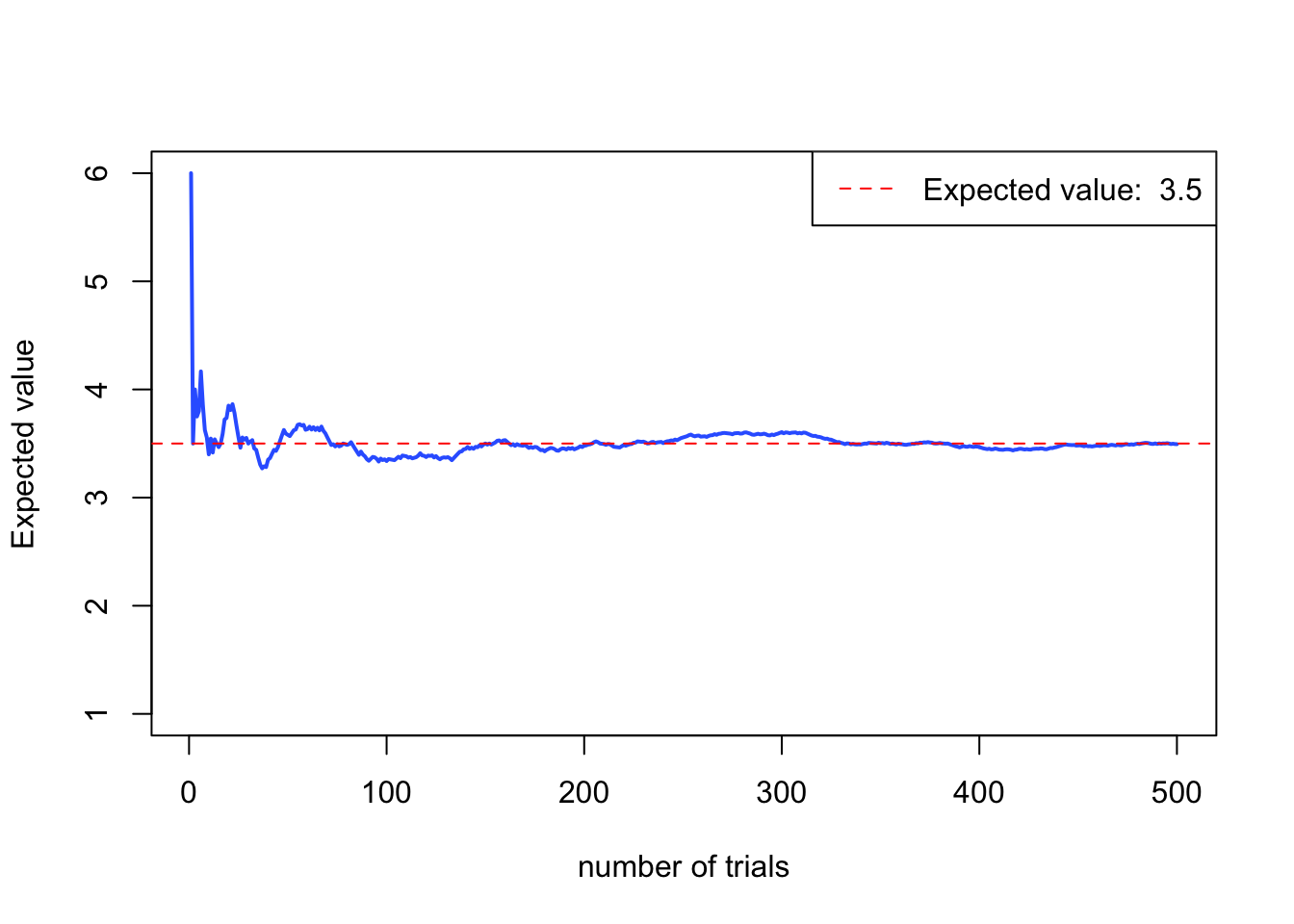#### Mean of a Discrete Random Variable

The mean of a discrete random variable $$X$$ is denoted $$\mu_X$$ or, when no confusion will arise, simply $$\mu$$. The terms expected value, $$E(X)$$, and expectation are commonly used in place of the term mean.

$E(X) = \sum_{i=1}^{N}x_iP(X=x_i)$

In a large number of independent observations of a random variable $$X$$, the $$E(X)$$ of those observations - the sample - will approximate the mean, $$\mu$$, of the population. The larger the number of observations, the closer $$E(X)$$ is to $$\mu$$ (Weiss 2010).

Let us recall our experiment from the previous section, when we picked 1,000 individuals and asked for the number of siblings. Let us again take a look at the table, summarizing the experiment

$\begin{array}{c|lcr} \text{Siblings} & \text{Frequency} & \text{Relative}\\ \ x & f & \text{frequency}\\ \hline 0 & 205 & 0.205 \\ 1 & 419 & 0.419 \\ 2 & 280 & 0.28 \\ 3 & 65 & 0.065 \\ 4 & 29 & 0.029 \\ 5 & 2 & 0.002 \\ \hline & 1000 & 1 \end{array}$

Let us calculate the expected value (mean) for that experiment.

\begin{align} \\ & E(X) = \sum_{i=1}^{N}x_iP(X=x_i) \\ & = 0 \cdot P(X=0) + 1 \cdot P(X=1)+ 2 \cdot P(X=2) + 3 \cdot P(X=3) +4 \cdot P(X=4)+ 5 \cdot P(X \ge 5) \\ & = 0 \cdot 0.205 + 1 \cdot 0.419 + 2 \cdot 0.28+ 3 \cdot 0.065 + 4 \cdot 0.029 + 5 \cdot 0.002 \\ & = 1.3 \end{align}

The resulting expected value of 1.3 is close to the mean $$\mu$$, which we calculate by using the population’s probabilities (real probabilities are taken from the lower right figure in the previous section).

$\mu = 1 \cdot 0.2 + 2 \cdot 0.425 + 3 \cdot 0.275 + 4 \cdot 0.07 + 5 \cdot 0.025 = 1.31$

##### Exercise

Let us consider a fair six sided dice. We can easily compute the expected value $$E(X)$$ using R. The term fair means that each random variable $$X=x_i,\; x \in 1,2,3,4,5,6$$ is equally likely to occur. Therefore $$P(X=x_i) = \frac{1}{6}$$.

$E(X) = \sum_{i=1}^{6}x_iP(X=x_i) = 1 \cdot \frac{1}{6} + 2 \cdot \frac{1}{6} + 3 \cdot \frac{1}{6} + 4 \cdot \frac{1}{6} + 5 \cdot \frac{1}{6} + 6 \cdot \frac{1}{6} = 3.5$

In R we write the following code:

# Expected value of a fair six sided dice
p.die <- 1/6
die <- c(1,2,3,4,5,6)
sum(die*p.die)
##  3.5

However, what if we are not sure if the dice is really fair? How to know that we are not cheated? Or to put it in other words: How often do we need to roll a dice before we can be more confident?

Let us do a computational experiment: We know from reasoning above that the expected value of a 6-sided fair dice is 3.5. We conduct an experiment by rolling a dice over and over again. We store the result and before we roll the dice again we calculate the average of all dice rolls so far. In order to achieve that little experiment, we write a for loop in R.

### Simulation ###
eyes <- seq(1,6) # possible events
probs <- rep(1/length(eyes),length(eyes)) # probabilities
expected.value <- round(sum(eyes*probs),2) # calculate expected value
n <- 500 # number of maximum rolls
values <- NULL # initialize an empty vector to store the value
averages <- NULL # initialize an empty vector to store the average values so far

#for-loop
for (roll in 1:n){
values <- c(values, sample(x = eyes, size = 1, prob = probs)) #sample method, type help(sample()) for further information
averages <- c(averages, mean(values))
}

### Plot ###
par(xpd = FALSE)
plot(x = seq(1:length(averages)),
y = averages,
type = 'l',
ylim = c(min(eyes), max(eyes)),
lwd = 2,
ylab = 'Expected value',
xlab = 'number of trials',
col = "#3366FF")
abline(h = expected.value, lty = 2, col = 'red')
legend('topright',
legend = paste("Expected value: ", as.character(expected.value)),
col = "red",
lty = 2)The graph shows that after some initial volatile behavior, the curve finally flattens and approximates the $$E(X)$$ of 3.5.

#### Standard Deviation of a Discrete Random Variable

The standard deviation of a discrete random variable $$X$$ is denoted $$\sigma_X$$ or, when no confusion will arise, simply $$\sigma$$. It is defined as

$\sigma = \sqrt{\sum_{i=1}^{N}(x_i-\mu)^2P(X=x_i)}$

##### Exercise

Let us turn to R and calculate the standard deviation for the dice roll experiment from above. During the experiment we rolled 500 times. The outcome of these rolls are stored in the vector values. The probability for each of these numbers in the vector values approximates $$\frac{1}{6}= 0.167$$. So we just put those numbers in the equation for the standard deviation from above. Remember, that the mean is stored in the expected.value variable.

x <- seq(1,6)
p.x <- prop.table(table(values))
p.x
## values
##     1     2     3     4     5     6
## 0.160 0.184 0.170 0.140 0.180 0.166
sqrt(sum((x-mean(values))^2 * p.x))
##  1.712882

Roughly speaking, our experiment showed that after 500 rolls, on average, the value of a dice number is 1.71 from the experimental mean of 3.494.